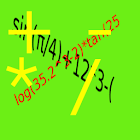All Android applications categories

All Android games categories# EQ Calc

by: 5 6

6 Users
rating

## Screenshots

Description

Equation entry/editable multi-function Calculator
Significant digit > 16
upper compatible to version 1.0
Capable to save equations/constants on SD card on Menu.

enter equation and Calculate.You can edit equation after many times.
If equation is too long.Touch equation and slide it.
Function calculation capable.
CLR:clear equation
BS:delete 1 char
Ans:set previous answer to equation
<>:enter edit mode and move cursor
ok:return to input mode
=:print answer

Tags: calculator , 2.2.119 , eq calc , eqcalc actions

from 5 reviews

"Good"

6